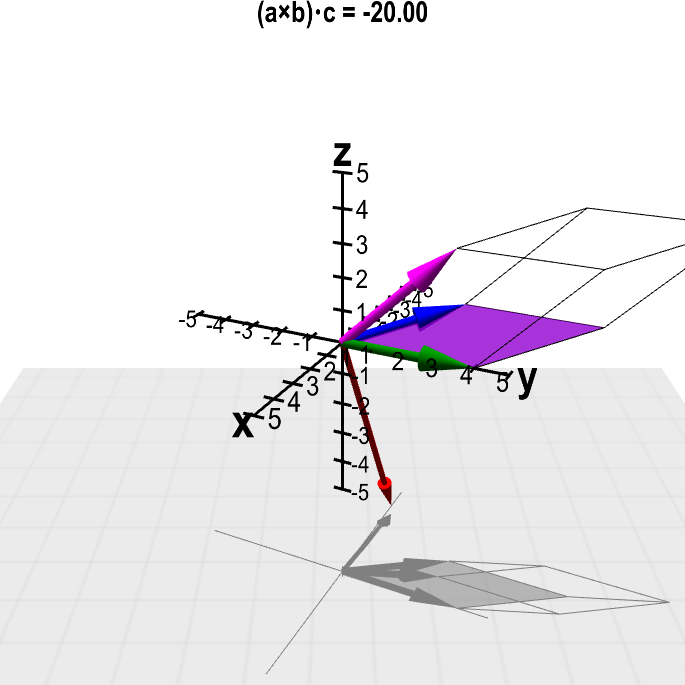# Math Insight

### Applet: Scalar triple product with fixed valuesThe cyan slider shows the value of the scalar triple product $(\color{blue}{\vc{a}} \times \color{green}{\vc{b}}) \cdot \color{magenta}{\vc{c}}$, where the vectors $\color{blue}{\vc{a}}$ (in blue), $\color{green}{\vc{b}}$ (in green), and $\color{magenta}{\vc{c}}$ (in magenta) are fixed to given values. The volume of the spanned parallelepiped (outlined) is the magnitude $\|(\color{blue}{\vc{a}} \times \color{green}{\vc{b}}) \cdot \color{magenta}{\vc{c}}\|$. The cross product $\color{blue}{\vc{a}} \times \color{green}{\vc{b}}$ is shown by the red vector.

The given vectors are $\color{blue}{\vc{a}} = (-2,3,1)$, $\color{green}{\vc{b}} = (0, 4, 0)$, and $\color{magenta}{\vc{c}} = (-1,3,3)$, so that scalar triple product is $(\color{blue}{\vc{a}} \times \color{green}{\vc{b}}) \cdot \color{magenta}{\vc{c}}=-20$.

The three-dimensional perspective of this graph is hard to perceive when the graph is still. If you keep the figure rotating by dragging it with the mouse, you'll see it much better. (Apologies to color blind people for reliance on colors.)